# 第三讲扩展,VA,RVA,FA(RAW),模块地址的概念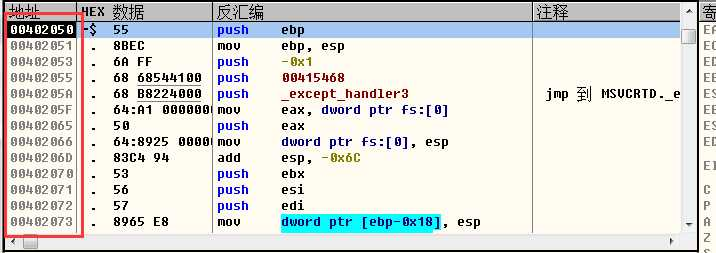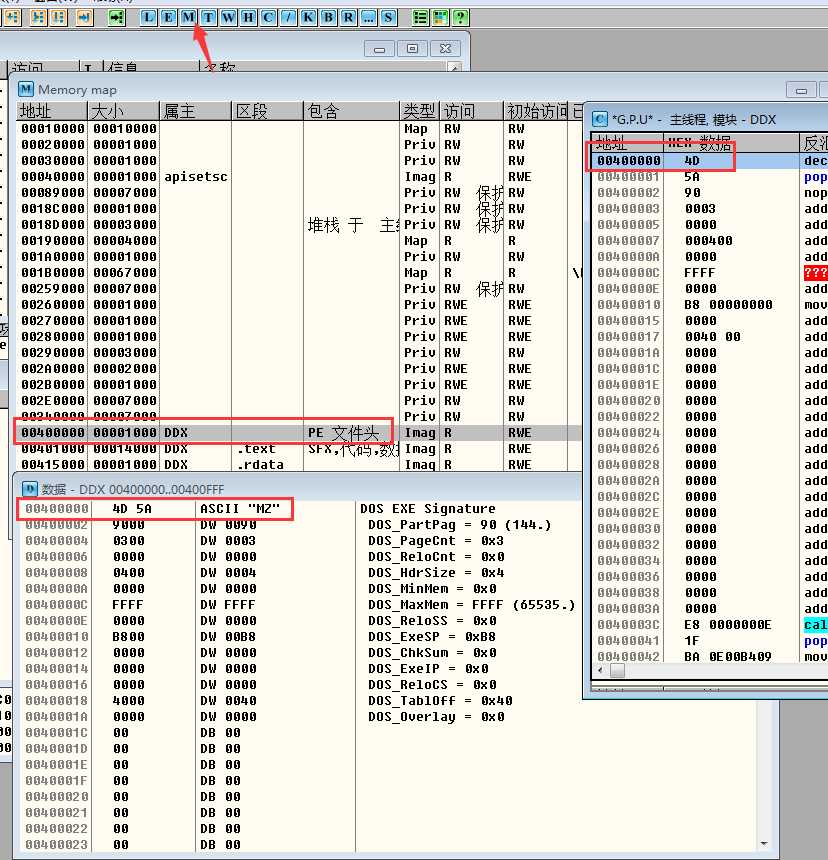VA = 0X4001200   (虚拟地址)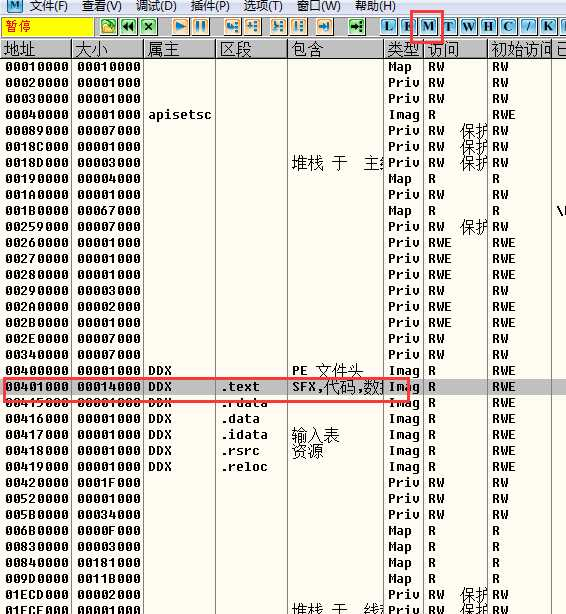RVA = VA - 401000  得出的就是相对于虚拟地址的偏移

RVA = 401200 - 401000 = 200(RVA)  那么偏移就是200了

FA就是文件中的地址.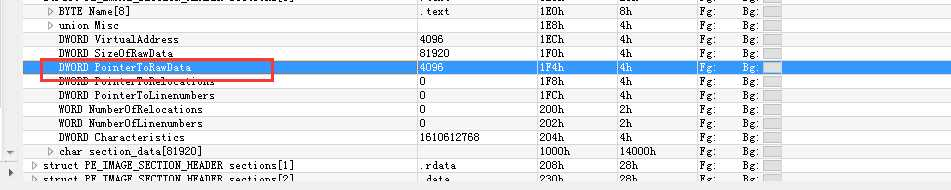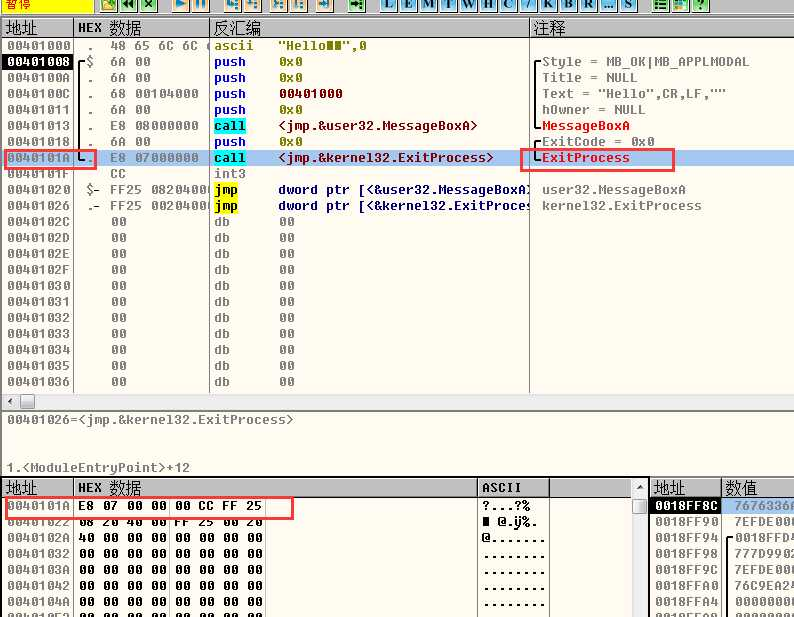1.获得虚拟地址(VA)  现在是40101A

2.查看属于哪个节区表(点击内存查看,OllyDbg)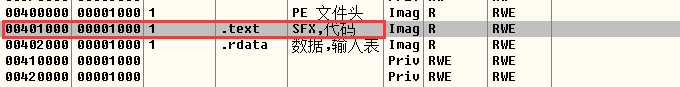3.算出RVA(相对虚拟地址偏移)

RVA = VA - 内存中节区地址

RVA = 40101A - 401000

RVA = 1A   (相对虚拟地址偏移是1A)

4.RVA + 文件中的(相同节表,比如上面是.text,那么文件中看的节表就是.text这个节表)节表中的PointerToRawData成员记录的大小得出虚拟地址在文件中的偏移

1A + (文件中节表的偏移) = 实际虚拟地址在文件偏移记录的代码地址.

1A + 200 = 21A  (虚拟地址在文件中的偏移)

200要查看节表,还记得上面我们算RVA的时候吗,找的是内存中节区的地址,而这个地址正好是.text代码区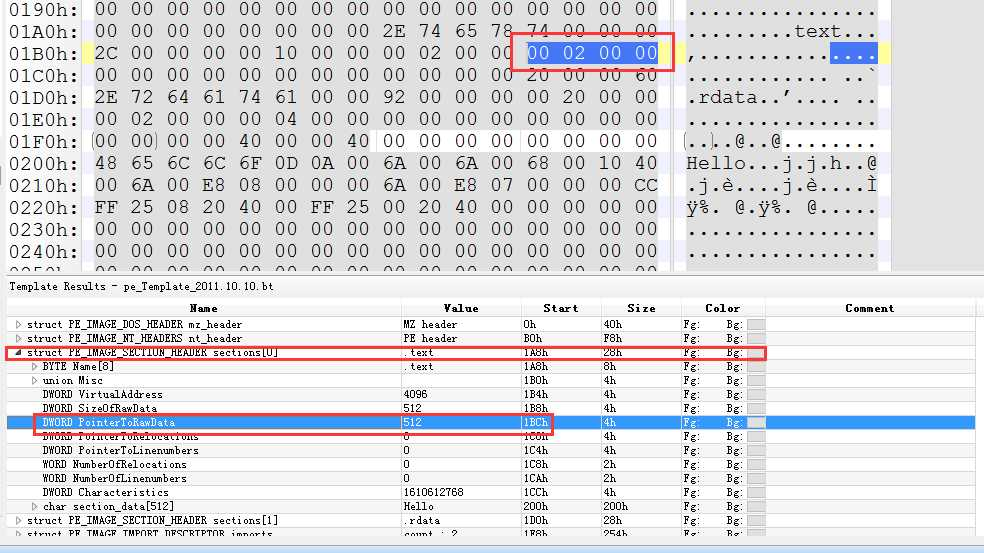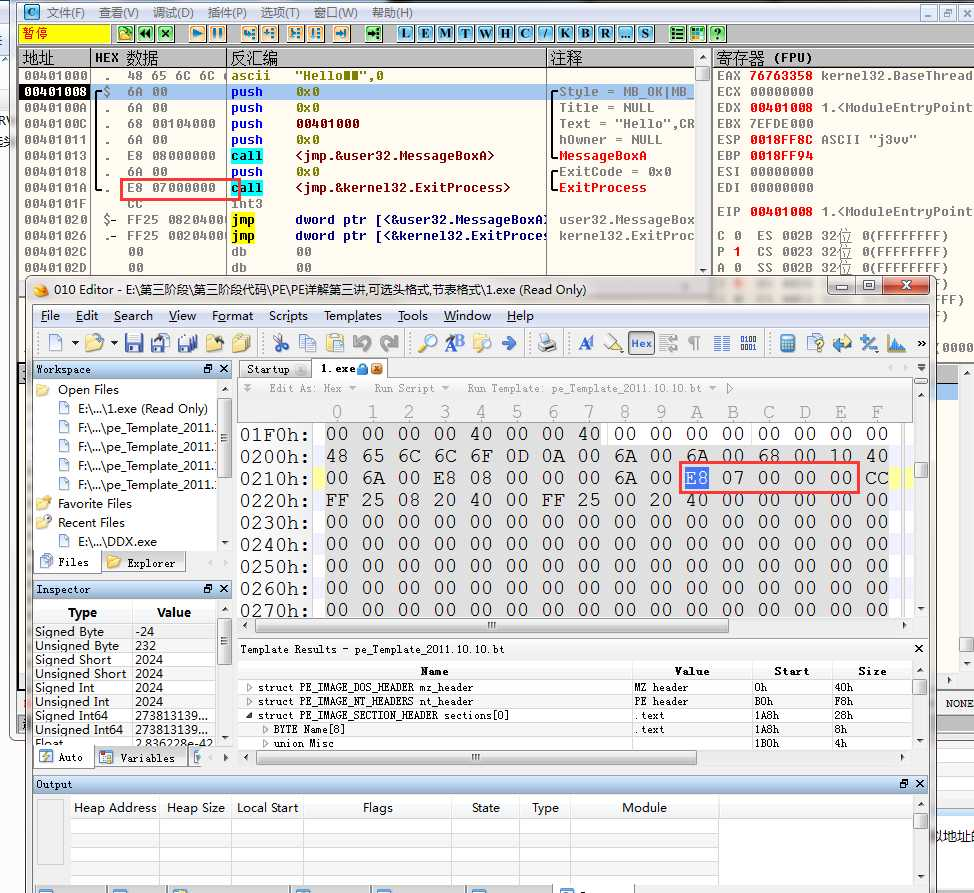其实很简单,首先看属于哪个节表的,  那么先算出RVA的值,然后让RVA + 文件中相同节表中的成员(PointRawData)  那么最终就是虚拟地址代码,在文件偏移的位置.

VA = 401456

RVA = 401456 - (.text的位置当然这个你得自己看,可能不是,这里默认是了)401000 = 456

FA = 456 + (文件中节表中的PointRawData,我假设是200,这里具体看PE中怎么存储的)200 = 656(十六进制)

VA = 401234

Image Bae = 400000

RVA = 401234 - 400000 = 1234

VPK  = (内存中节区首地址 - image base) - 文件中节区的偏移地址(PointerToRawData 字段)

(401000 - 400000 ) - 400(这个值自己看文件,不一定是400)= 1000 - 400 = C00(vpk);

FA = RVA - VPK  = 1234 - C00 = 634

RVA = 401456 - 00400000 = 1456

VPK = (401000 - 400000) - 文件中PointerToRawData 字段

=  1000 - 400 = C00

FA = RVA - VPK

= 1456- C00 = 856

FA = 　VA - 内存中节区地址 + 文件PointerToRawData 字段

401596 - 401000 + 400

= 596 + 400

= 996  (FA)

VA = 401596

IMAGEbase = 400000

RVA = (虚拟地址 - 模块地址)

=401596 - 400000

= 1596

VPK = (节区表首地址- 模块地址)  - 节表中的文件PointerToRawData 字段

= 401000 - 40000 - 400

= 1000 - 400

=　C00 (vpk)

FA = RVA - VPK

= 1596 - C00

=  996

1.你要知道一个文件位置, (随便哪个都行,把它转换为内存虚拟地址)

2.我们要知道 文件偏移位置的大小,(也就是上面说的节表中的  PointerToRawData 字段)

3.我们要知道你给的文件位置属于哪个区,这个是根据 上面计算出来FA的首地址的出来的

VA = FA +imagebase(模块首地址) + VPK

VPK的值就是你要计算的

VPK = (内存中的节区表 - 模块地址) - PointerToRawData字段

VA = 996 + 40000 + (401000 - 400000 - 400)

=   40996 + C00

=   41596 (虚拟地址位置)

(0)
(0)